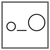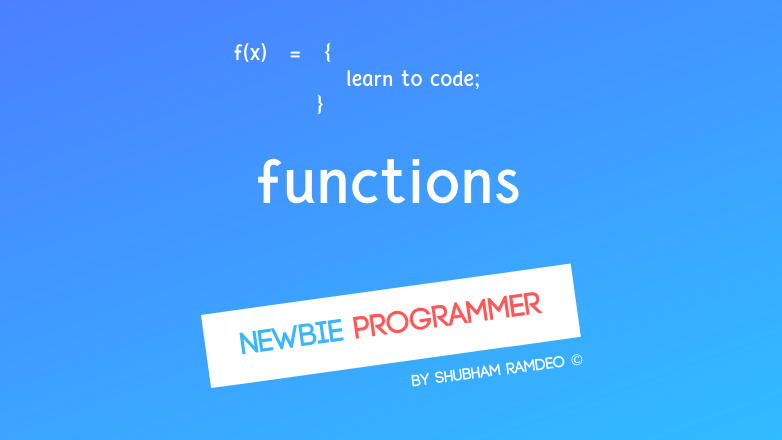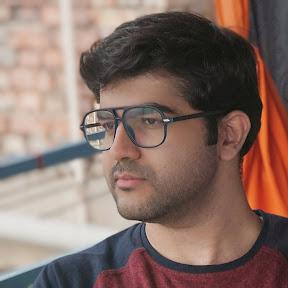Fuddlepixel coz tech is fun();

# Functions

Let us discuss what are functions and how to use them in C language. How functions can reduce our work and can make our programming worth !

By Shubham Ramdeo
07 October 2015 · 6 mins read
# `c`  `newbie`That’s how a program can be divided into functions and can be called and reused in many ways !

Welcome to the Newbie Programmer Series. In the last post we had an introduction with the program structure. In this post, we will discuss functions. If you have not read that previous part then don’t worry I have the introduction here too. So if you are new to this series, please go to the index (click here) and read out all the previous parts so that you can easily understand what is going down below.

This is a big post, involves many concepts, please try a second reading or contact me if you didn’t get it at once. Please continue reading below.

## Introduction

It’s easy for those who know maths, but don’t worry I am here. Function means a specified work. For example, what is the function of a switch ? Its to turn the appliance ‘on’ or ‘off’. Or, What is the function of the ‘jump’ button in a video game ? Its to make your hero jump. To each of these things, there is assigned a specific work to do. That is a function. In mathematical sense, you can understand a function as a formula. For example :

f (x) = 3x

This is a very simple function. The function is ‘f’ . It takes the value of x _and then the specific work is to multiply that _x _ by 3. It is not necessary to have some given value _x . For example the function g() = 4 Will always give value 4 Or can make functions with many variables like add (x , y) = x + y Programming is not only about maths, try to think different ways through which you can complete some of your work. Lets take an example of a bulb switch : switch ( button ) { if  button = on, then turn the bulb ‘on’ if button = off, then turn the bulb ‘off’ } Now that is a function too. So to turn a bulb on, just command “switch ( on )” ! Well coding is easy, the hard part is to make concept of a function. Anyways one should think how he can break his problem into simpler problems. That will help him to design a function just as we did above. NOTE:-_ There is a little more theory below. It may look awkward but please read it and after that coding stuff is down below and that will make your confusion clear. _

## Variable data type

Now this is a little tricky topic but I will try to keep everything simple and easy. Before making any function during programming, we have to specify what type of information the function would handle.

For an example, the function of bulb switch above have only two type of information. Its ‘on’ or ‘off’. To represent them, we can use ‘1’ or ‘0’. Therefore basically we are using integers for this function as 0 and 1 are integers.

Now read the above parts again.

In C Language, we have already discussed we have many types of variables. They are integers ( int ) or Point decimals ( float ) or characters ( char ). And so we have to specify what type of variable the function is using.

Like A function multiplying two integers : muly ( x, y ) = x * y has to specify int x, y.

If that is involving point decimals too, then we have to write float x, y.

## Function returning data type

Just as we specify the type of variables involved, we also have to specify the type of value the function is returning. For example, the above muly ( x, y ) function for multiplication involves two integers x and y but after multiplying x and y, it will return the product. That product will also be integer. And that makes the function muly itself an integer function.

## Void

When the function or variable is not an integer, character etc, I mean when the function has no specific information type, we use the word void. So the functions which take no values or return no values are always considered void functions.

## Return

We are continuously using the word ‘return’ again and again in our codes but never discussed about it. Lets do it here.

This ‘return’ is usually used at the end of a function and it returns the result of the function.

So if in the above multiplication function, if C = x * y, then we will write return(C) to give the result of the function.

### return 0

What we have used so far is return 0, which means there is no error and the program is completed successfully And it exits !

Lets do the coding stuff :

## Defining a Function in C

Its simple, just get the types and write as :

``````function-type function-name ( variable-type variable names )
{
// function body
// return ( returning variable )  (optional)
}
``````

In old times, the above thing was written as :

``````variable-types variable names
function-type function-name ( variable names )
{
// function body
// return ( returning variable )  (optional)
}
``````

But both are correct.

``````int add ( int x, int y )
{
int c;
c = x + y;
return ( c );
}
``````

More easy, just write :

function-name( variable values if involved );

note the semicolon.

So you can use the add function in your program as :

## Practically :

Lets make the addition function in different ways :

``````#include<stdio.h>
main()
{
int sum;
sum = add ( 3, 4 );
printf("Sum of 3 and 4 is %d", sum);
}

int add ( int x, int y )
{
int c;
c = x + y;
return (c);
}

#include<stdio.h>
main()
{
printf("Sum of 3 and 4 is %d", add(3,4) );
}

int add ( int x, int y )
{
int c;
c = x + y;
return (c);
}

#include<stdio.h>
main()
{
printf("Sum of 3 and 4 is %d", add(3,4) );
}

int x, y;
{
int c;
c = x + y;
return (c);
}

#include<stdio.h>
main()
{
printf("Sum of 3 and 4 is %d", add(3,4) );
}

int x, y;
{
return ( x + y );
}
``````

All of the above four programs are exactly the same. And have the same output :

``````Sum of 3 and 4 is 7
``````

And the functions didn’t ends here, we have discussed very little yet, there are many important concepts tricks and tips that we will be discussing.  We did a very simple mathematical function but what about other types ? specially the void one ? We will also discuss different ways of using functions. But for the sake of page limit, We will discuss it in the next post. So stay connected !

Please share this post as much as you can so that we can get connected to more geeks who wants to learn programming but have no clue. Thanks for reading :)

##### Update me weekly ✉

Subscribe below and we’ll send you a weekly email summary of all new Code tutorials. Never miss out on learning about the next big thing.

#### Shubham RamdeoShubham Ramdeo is the founder of Fuddlepixel. He is a geek, a writer, involved with many technical and humanity projects.

Meet me!

#### Recent#### Related

`c`

`newbie`

Top